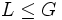# Intermediately characteristic subgroup

BEWARE! This term is nonstandard and is being used locally within the wiki. [SHOW MORE]
This article defines a subgroup property: a property that can be evaluated to true/false given a group and a subgroup thereof, invariant under subgroup equivalence. View a complete list of subgroup properties[SHOW MORE]
This is a variation of characteristicity|Find other variations of characteristicity | Read a survey article on varying characteristicity

## Definition

### Symbol-free definition

A subgroup of a group is said to be intermediately characteristic if it is characteristic not only in the whole group but also in every intermediate subgroup.

### Definition with symbols

A subgroup$H$ of a group$G$ is said to be intermediately characteristic if for any intermediate subgroup$K$ (such that$H \le K \le G$),$H$ is characteristic in$K$.

## Formalisms

### In terms of the intermediately operator

This property is obtained by applying the intermediately operator to the property: characteristic subgroup
View other properties obtained by applying the intermediately operator

The subgroup property of being intermediately characteristic can be obtained by applying the intermediately operator to the subgroup property of being characteristic.

## Examples

VIEW: subgroups of groups satisfying this property | subgroups of groups dissatisfying this property
VIEW: Related subgroup property satisfactions | Related subgroup property dissatisfactions

## Relation with other properties

### Stronger properties

Property Meaning Proof of implication Proof of strictness (reverse implication failure) Intermediate notions
order-unique subgroup finite subgroup and no other subgroup of the same order (via isomorph-free) (via isomorph-free) Isomorph-free subgroup|FULL LIST, MORE INFO
isomorph-free subgroup no other isomorphic subgroup (obvious) any non-co-Hopfian group, such as the group of integers, as a subgroup of itself Intermediately injective endomorphism-invariant subgroup, Isomorph-containing subgroup|FULL LIST, MORE INFO
isomorph-containing subgroup contains every isomorphic subgroup Intermediately injective endomorphism-invariant subgroup|FULL LIST, MORE INFO
homomorph-containing subgroup contains every homomorphic image Intermediately fully invariant subgroup, Intermediately injective endomorphism-invariant subgroup, Intermediately strictly characteristic subgroup, Isomorph-containing subgroup|FULL LIST, MORE INFO
subisomorph-containing subgroup contains every subgroup isomorphic to a subgroup of it Isomorph-containing subgroup, Transfer-closed characteristic subgroup|FULL LIST, MORE INFO
intermediately fully invariant subgroup fully invariant in every intermediate subgroup Intermediately injective endomorphism-invariant subgroup, Intermediately strictly characteristic subgroup|FULL LIST, MORE INFO
intermediately injective endomorphism-invariant subgroup injective endomorphism-invariant in every intermediate subgroup (obvious) |FULL LIST, MORE INFO
transfer-closed characteristic subgroup intersection with any subgroup is characteristic in that subgroup transfer-closed characteristic implies intermediately characteristic intermediately characteristic not implies transfer-closed characteristic |FULL LIST, MORE INFO

### Weaker properties

Property Meaning Proof of implication Proof of strictness (reverse implication failure) Intermediate notions
intersection of finitely many intermediately characteristic subgroups intersection of finitely many intermediately characteristic subgroups (obvious) follows from intermediate characteristicity is not finite-intersection-closed |FULL LIST, MORE INFO
sub-intermediately characteristic subgroup there is a chain of subgroups from the subgroup to the whole group, with each member intermediately characteristic in its successor (obvious)) follows from intermediate characteristicity is not transitive |FULL LIST, MORE INFO
characteristic subgroup invariant under all automorphisms (obvious) characteristicity does not satisfy intermediate subgroup condition Intersection of finitely many intermediately characteristic subgroups|FULL LIST, MORE INFO
normal subgroup invariant under all inner automorphisms (conjugations) (via characteristic) (via characteristic) Characteristic subgroup|FULL LIST, MORE INFO

## Metaproperties

BEWARE! This section of the article uses terminology local to the wiki, possibly without giving a full explanation of the terminology used (though efforts have been made to clarify terminology as much as possible within the particular context)

Here is a summary:

Metaproperty name Satisfied? Proof Difficulty level (0-5) Statement with symbols
transitive subgroup property No intermediate characteristicity is not transitive It is possible to have groups$H \le K \le G$ such that$H$ is intermediately characteristic in$K$ and$K$ is intermediately characteristic in$G$ but$H$ is not intermediately characteristic in$G$.
quotient-transitive subgroup property Yes intermediate characteristicity is quotient-transitive Suppose we have groups$H \le K \le G$ such that$H$ is intermediately characteristic in$G$ and$K/H$ is intermediately characteristic in$G/H$. Then,$K$ is intermediately characteristic in$G$.
intermediate subgroup condition Yes (obvious) 0 Suppose$H \le K \le G$ are groups such that$H$ is intermediately characteristic in$G$. Then,$H$ is intermediately characteristic in$K$.
finite-intersection-closed subgroup property No intersection of two isomorph-free subgroups need not be intermediately characteristic It is possible to have a group$G$ and intermediately characteristic subgroups$H, K$ of$G$ such that$H \cap K$ is not intermediately characteristic in$G$ (i.e., there exists$L \le G$ containing$H \cap K$ as a non-characteristic subgroup). In fact, we can choose an example where both$H$ and$K$ are isomorph-free in$G$.
strongly join-closed subgroup property Yes intermediate characteristicity is strongly join-closed Suppose$H_i, i \in I$ is a family of intermediately characteristic subgroups of a group$G$. Then, thee join of subgroups$\langle H_i \rangle_{i \in I}$ is also intermediately characteristic in$G$.

## Effect of property operators

### Right transiter

It turns out that any intermediately characteristic subgroup of a transfer-closed characteristic subgroup is again intermediately characteristic. This follows from some simple reasoning and the fact that characteristicity is itself transitive. Further information: Intermediately characteristic of transfer-closed characteristic implies intermediately characteristic

Hence, the right transiter of the property of being intermediately characteristic is weaker than the property of being transfer-closed characteristic.